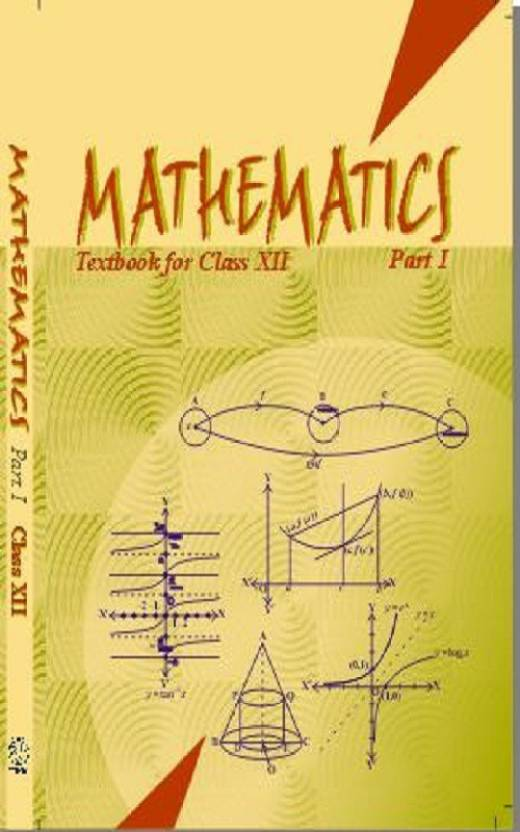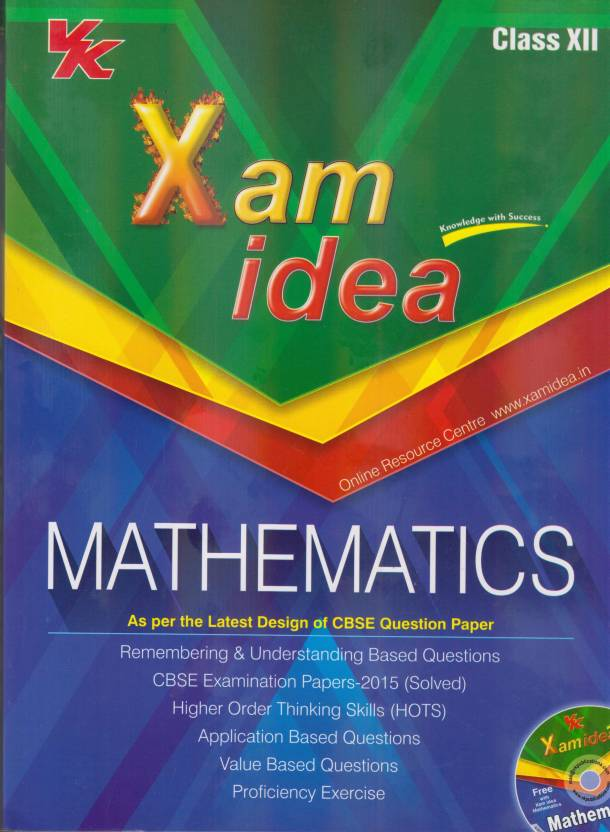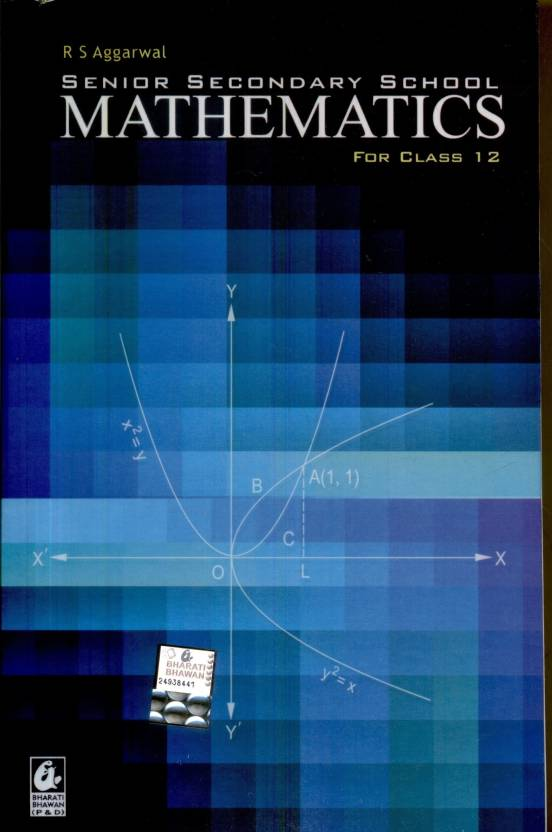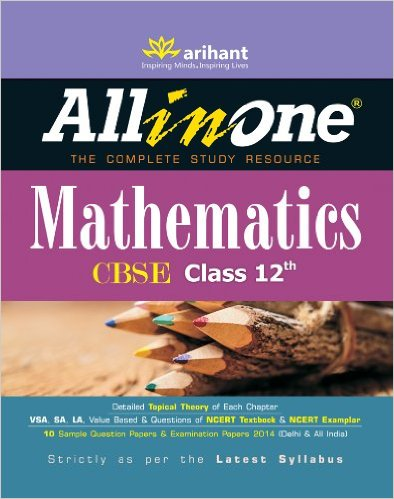# CBSE Class 12 : BEST Reference Books For Mathematics To Get 90+## NCERT Mathematics Class 12th Part - I• The book provides covers a variety of important and fundamental topics in math for the 12th grade.
• Every chapter contains detailed theory supported by illustrations, tables, flow charts, algorithms, notes, and remarks, solved examples, worked out problem.
• The questions given after each chapter are very important for CBSE Board Exam.
• Practice exercise at the end of each chapter.
• Book have been set keeping the CBSE curriculum and exam patterns in mind.
• This book is the NCERT recommended guide for those eligible applicants of the NTSE and NAS.

• ## NCERT Mathematics Class 12th Part - II• The book provides covers a variety of important and fundamental topics in math for the 12th grade.
• Every chapter contains detailed theory supported by illustrations, tables, flow charts, algorithms, notes, and remarks, solved examples, worked out problem.
• The questions given after each chapter are very important for CBSE Board Exam.
• Practice exercise at the end of each chapter.
• Book have been set keeping the CBSE curriculum and exam patterns in mind.
• This book is the NCERT recommended guide for those eligible applicants of the NTSE and NAS.

• ## NCERT Solutions Mathematics 12th• The book proved very useful for aspirants preparing for different competitive entrance examinations.
• Every chapter contains detailed theory supported by illustrations, tables, flow charts, algorithms, notes, and remarks, solved examples, worked out problem.
• step by step approach for tackling each topic, explaining difficult problems with solutions that can be approached by more than one formulae.
• Book have Questions that were asked in Previous Years’ Examinations from 2008-2015.
• Book have been set three Practice Sets that are included at the end of each section.
• Solutions along with appropriate explanations have been given.

• ## Xamidea Mathematics for Class - 12• The book proved Remembering and Understanding Based Question.
• CBSE Examination paper 2015 (SOLVED).
• Higher order thinking Skills (HOTS)
• Application based question.
• Value based question.
• proficiency exercise.

• ## Mathematics Class - 12 (Set of 2 Volumes) R.D. Sharma• The book has been designed by experts in the subject and this makes the book highly reliable to the Students.
• The solutions are well arranged according to their chapters.
• R.D. Sharma has included numerous graded solved examples.
• R.D. Sharma has brief summary of the topics discussed.
• Book help students quickly revise important formulae and concepts.
• Students will also come across many exercises which contain unsolved multiple choice questions (MCQs).

• ## R.S. Aggarwal Senior Secondary School Mathematics for Class - 12• Comprehensive guide for those taking the twelfth standard CBSE examinations.
• All the topics that are required as per syllabus are covered, step by step.
• A number of other topics are explained in great detail.
• One of the important features of this book is that problems are graded.
• After each batch of solved examples, there are comprehensive unsolved exercises.
• Also for students who are preparing for competitive examinations.

• ## ALL in One Mathematics CBSE class 12• The book provides guidance to the students starting from the stage of learning.
• Every chapter contains detailed theory supported by illustrations, tables, flow charts, algorithms, notes, and remarks, solved examples, worked out problem.
• The questions given in each chapter have been grouped as very short answer type, short answer type and long answer type questions.
• Practice exercise, a small test that has been given at the end of each chapter.
• 10 sample question papers have been given after the chapter wise study.
• Toppers answer sheet have been given at the end of the book.